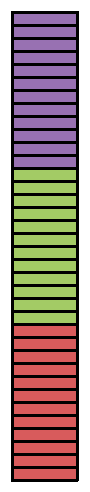### Home > CC1 > Chapter 9 > Lesson 9.2.1 > Problem9-38

9-38.

Express each of the following lengths in feet.

Remember that $12$ inches are equal to $1$ foot and $3$ feet are equal to $1$ yard. These conversions will come in handy!

1.  $24$ inches

1.  $18$ inches

1.  $9$ inches

1.  $2$ yards

1.  $3$ inches

1.  $2\frac{1}{2}$ yards

Try drawing a diagram of how many inches are in a foot to compare to each of these measures.

For instance, James converted $36$ inches to feet by drawing the diagram at right. He could see that there are $3$ groups of $12$ inches in $36$ inches, so that means $36$ inches is equal to $3$ feet!

If one yard is $3$ feet, how many feet are in $2$ yards? Can you use multiplication? Why would that make sense?

$3\text{ inches }=\frac{1}{4}\text{ feet.}$
Don't forget to show your work.

Use your answer from part (d), as well as the hint provided to help you through this problem.

$2\frac{1}{2}\text{ yards }=7\frac{1}{2}\text{ feet.}$